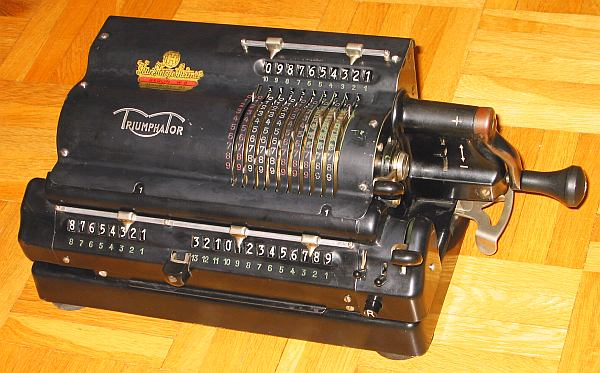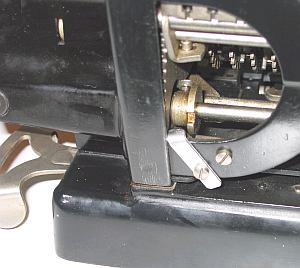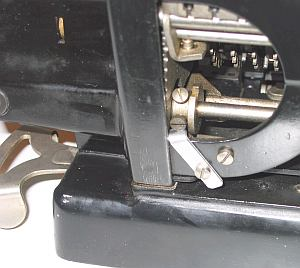previous <<==>> next

TRIUMPHATOR   CRN1Model CRN1 has a BACK-TRANSFER Button »R« right on the carriage

 ```TECHNICAL DATA of the Mechanical Calculator TRIUMPHATOR CRN1 ***************************************************************************** Serial Number: 231339 Dimensions: (ca.) Width = 13_1/2" / 34 cm Depth = 5_1/2" / 14 cm Height = 5_1/2" / 14 cm Weight: (ca.) 14 lbs / 6.5 kg Mechanics: Pin-Wheel / Sprossenrad 10s Carry Mechanism Functions: Add, Subtract, Multiply, Divide Back-Transfer / Rueckuebertragung Registers: Input = 10 Decimals Counter = 8 Decimals Arithmetic = 13 Decimals Manufacturer: TRIUMPHATOR-WERK Moelkau b. Leipzig East Germany 1955 H O W T O U S E the TRIUMPHATOR CRN1 ******************************************** Same procedure as with Model CN1 ... ... Except BACK-TRANSFER possibility. BASIC SETTINGS: =============== (a) Crank: ---------- For addition (+) the crank is turned clockwise; for subtraction (-) the crank is turned counter-clockwise. In the idle position the crank is DOWN & LOCKED. To make one or more turns with the crank, pull out the handle to unlock. When finished the turn(s), let the handle snap into lock again. The locked down position only makes other functions (ex. clearing) accessible. REMARK: Every started turn has to be finished completely! Accidentally started turns are correctible somehow. (b) Clearing Registers: ----------------------- The input register can be cleared with the upper right lever by lifting it up (It is intended to use the thumb for). The arithmetic register & the counter unit will be cleared with the big lever on the right side of the carriage by lifting it up. Depending of the two little switches left of it, both registers can be cleared simultaniously (when both switches are down) or separate (when up = locked; left = counter, right = arithmetic unit). (c) Shifting the Carriage: -------------------------- With the 2-grip lever (located under the crank) the carriage is movable one step right or one step left. With the front button the carriage can be moved continuously when pressed down and right or left. The left position "1" is the "Start Position" of the carriage. (d) Counting Direction: ----------------------- With the (+ <==> -) switch on the crank's holder it enables the counter to work positive with (+) positive (clockwise) turns - and positive too with (-) negative (counter-clockwise) turns of the crank. (e) Back Transfer: ------------------ For continuous multiplication the contents of the arithmetic unit can be back transfered into the input register with this procedure: (1) Clear Input Register, (2) Push »R« Button, (3) Clear Arithm.Unit. In case the »R« Button is pressed accidentally, the error can be corrected: LIFT both little LOCK-SWITCHES and "CLEAR" Arithm.Unit. ADDITION & SUBTRACTION: ======================= Example: 123 + 45 - 6 = 162 Clear input, counter and arithmetic units; counter switch to (+) ADD: Enter the first number (123) in the far right of the input unit. Make a positive (clockwise) turn with the crank to transfer the number into arithmetic unit. The counting unit displays the figure 1. Enter the second number (45). Make a positive (clockwise) turn with the crank to add the number. The arithmetic unit displays the intermediate sum (168) and the counting unit displays the figure 2. SUBTRACT: Enter the third number (6). Make a negative (counter-clockwise) turn with the crank. The arithmetic unit displays the result (162) and the counting unit is decreased by 1. REMARK: NEGATIVE RESULTS are displayed in the arithmetic unit as the COMPLEMENT of the next higher 10, 100, 1000, ... Example: -12 = 99...9988 MULTIPLICATION: =============== Example: 123 x 45 = 5535 Clear input, counter and arithmetic units; counter switch to (+) Enter the multiplicand (123) in the far right of the input unit. The multiplicator (45) has two digits, so the carriage is shifted to position 2 by pressing the 2-grip lever forward. Make positive (clockwise) turns with the crank, until the first figure of the multi- plicator (4) will appear in the 2nd position of the counter unit. Shift the carriage to position 1 by pressing the 2-grip lever backward. Repeat making positive turns with the crank, until the second figure of the multiplicator (5) appears in the 1st position of the counter unit. The multiplication is done: The multiplicand (123) stays in the input unit, the multiplicator (45) in the counter and the result (5535) is in the arithmetic unit. DIVISION: ========= Example: 22 : 7 = 3.1428571 Remainder 3 Division requires 3 steps: (1st) To Set the Dividend into Arithmetic Unit: ----------------------------------------------- For the maximum number of decimals, pull out the carriage to the far right position. Enter the dividend (22) in the far right of the input unit. Make a positive (clockwise) turn with the crank to transfer into arithmetic unit. (2nd) To Set the Divisor into Input Unit: ----------------------------------------- Clear counter & input units. Set counter switch to (-). Enter the divisor (7) above the dividend (22). (3rd) To Divide: ---------------- Make negative (counter-clockwise) turns with the crank until the arithmetic unit shows an "underflow". Make one positive (clockwise) turn with the crank. Move the carriage to the next left position. Repeat this procedure until the required number of decimals ... The result (3.1428571) is in the counter unit, and the remainder (3) is in the arithmetic unit. The divisor (7) stays in the input unit, therefore an additional decimal can be estimated... ( 4, cause 4 x 7 = 28). R E M A R K S T O T H E R E S T A U R A T I O N : ============================================================================= As usual, the CRN1 came in a not working state. After cleaning and oiling two ERRORS resisted ... (1) Only the (-) COUNTING-DIRECTION was working. When switched to (+) the crank was blocked. The analysis showed, switching to (+) resulted in a over-turn of the "Locking Device" (what is connected to the reverse-gear) A SENSOR PIN MISSED ITS GAP. Simple SOLUTION: Installation of an aluminum piece to stop the "Locking Device" in the right angle ...(-) Position (+) Position (2) Problems with CARRIAGE SHIFT - Same as with the TRIUMPHATOR CN1 ... ... and solved in the same way! impressum: ***************************************************************************** © C.HAMANN http://public.BHT-Berlin.de/hamann 10/05/08 ```